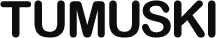Pages: 1 2 3 4 5 6

# Clumpy Usage Guide

## Indicating Progress

When a long-running operation is something the user cares about, it’s nice to provide the user with some indication of how close the operation is to completion. To this end, Clumpy lets you specify a function to be called between clumps of execution.

To specify this function, assign it as the `between` property of an object, and pass that object either to the `Clumpy` constructor, or to the `set` method of a `Clumpy` instance.

The following examples suppose that you have a function called `output` that inserts text into some element on the page. Here’s an example of the first way, passing it to the constructor.

``````var clumpy, i;

clumpy = new Clumpy({
between: function () {
output('Progress: ' + i);
}
});

clumpy.
for_loop(
function () { i = 0; },
function () { return i < 100000; },
function () { i += 1; },
function () {
// statements
}
);``````

Here’s an example using `set`, in which two consecutive loops use different progress indicators:

``````var i,
clumpy = new Clumpy();

clumpy.
set({
between: function () {
output('First Loop: ' + i);
}
}).
for_loop(
function () { i = 0; },
function () { return i < 100000; },
function () { i += 1; },
function () {
// statements
}
).
once(function () {
output('First Loop is Finished');
}).
set({
between: function () {
output('Second Loop: ' + i);
}
}).
for_loop(
function () { i = 0; },
function () { return i < 100000; },
function () { i += 1; },
function () {
// statements
}
).
once(function () {
output('Second Loop is Finished');
});``````

It produces something like this:

``````First Loop: 11664
First Loop: 23337
First Loop: 35012
First Loop: 46749
First Loop: 58401
First Loop: 70103
First Loop: 77520
First Loop: 89151
First Loop is Finished
Second Loop: 663
Second Loop: 11528
Second Loop: 22448
Second Loop: 33603
Second Loop: 44707
Second Loop: 55606
Second Loop: 66725
Second Loop: 77791
Second Loop: 88744
Second Loop: 99765
Second Loop is Finished``````

The `set` method behaves like `once`, enqueuing the act of setting `between` until everything before it is complete. It can also accept other options, which are described in the reference.

Next Page: Nesting